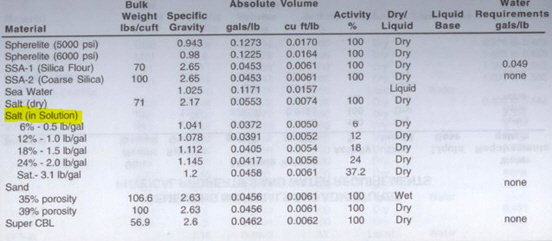# Salt Cement Systems

Some cement formulas have salt as NaCl, and KCl into the cement system and we call them “salt cement systems”. The salt cement systems cause confusion in cement calculations because salt can be dissolved in based fluid and the volume of salt in solid state is not the same as salt in solution phase. Therefore, density of solid salt is not the same as the dissolved salt in liquid.

To get correct density and absolute volume of salt solution, you can find information about from cementing hand books. The books should tell you how much % salt is in solution and how much absolute volume/density is. Please see an image below that I just got from my Halliburton redbook.The percentage (%) of salt is typically by weight of water. For example, 12% salt (NaCl) means 12% by weight of water.

The calculation is based on each cementing handbook so you may have slightly differences on the figures depending on which books you use. Anyway, the difference is usually insignificant.

We will go through one sample calculation so you can get idea how to work on it.

Cement formula:

Class G cement

35% Silica sand

18% salt (NaCl)

0.1 gps of chemical A

Required cement density = 16.0 ppg

The absolute volume of each material is listed below:

Class G cement = 0.0382 gal/lb

Silica sand = 0.0456 gal/lb

Chemical A = 0.0974 gal/lb

18% salt (NaCl) in solution = 0.0405 gal/lb (based on the Halliburton cementing handbook)

Determine yield of cement from this formula

Volume = Weight x Absolute Volume

Where:

Volume in gal/sack

Weight in lb/sack

Absolute volume in gal/sack

Firstly, we need to know how much volume of water.

The table below shows each component of cement slurry as shown below:

 Weight (lb/sack) Absolute volume (gal/lb) Volume (Gal/sack) 1 sack of class G cement 94 0.0382 94 x 0.0382 = 3.591 35% Silica sand 0.35 x 94 = 32.9 0.0456 32.9 x 0.0456 = 1.5 Chemical A 0.1 ÷ 0.0974 = 1.0267 0.0974 0.2 Water 8.33k 1÷8.33 k 18% Nacl (solution) 0.18 x 8.33k 0.0405 0.0405 x 0.18 x 8.33k Total 94+32.9+1.0267+8.33k+1.4994k 3.591+1.5+0.2+k+0.0607k

k is a variable that we need to solve.

Total weight per sack of cement =94+32.9+1.0267+8.33k+1.4994k = 127.9267 + 9.8294k

Total volume of cement slurry per sack of cement= 3.591+1.5+0.2+k+0.0607k = 5.291 + 1.0607k

Cement Density = Total weight per sack of cement ÷ Total volume of cement slurry

16.0 = (127.9267 + 9.8294k) ÷ (5.291 + 1.0607k)

Solve for k: k = 6.059

We can determine yield of cement as follows:

Yield of cement (Total volume of cement slurry per sack of cement) = 5.291 + (1.007 x 6.059) = 11.39 gal/sack

Ref books:Cementing Technology Books

Share the joy1.Mohamad Dangou says: